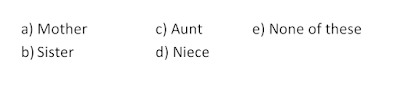Come out of the Dilemma: Switch To Government Jobs# Blood Relation II

In my last blog, we have discussed the Direct form of questions that actually not tough to resolve, but things can be harder as well. To make you people confuse, the examiner can ask in an indirect way to ensure your skills and smartness.

In Blood Relation, another type that is randomly asked in exams is “Form of Arithmetic puzzles.” Let’s take a problem:

Solve according to these following conditions:

A+B means A is the Daughter of B; A*B means A is the son of B; A-B means A is the wife of B then
1. If P*Q-S, Which is the following is true?

2. Solution:

First, check what you have given and make it in symbolic form to understand the right format as:
• P*Q implies P is the son of Q.
• Q-S implies Q is the wife of S.

Now match every option with a diagram:
1. S is the wife of Q = No, S is the husband of Q.
2. Q is the father of P = No, Q is the mother of P.
3. P is the daughter of Q = No, P is the son of Q.
4. S is the father of P = Yes, This is the right option.

Therefore, the option “d” is correct.
3. if T-S * B-M, Which of the following is not true?

4. Solution:

First, check what you have given and make it in symbolic form to understand the right format as:
• T-S implies T is the wife of S.
• B-M implies B is the wife of M
• S*B implies S is the son of B

Now match every option with a diagram:

** Note** Question is which of the following is not true means you have to choose one that is wrong.

1. B is the mother of S = Yes, it's true.
2. S is the daughter of B = No, it’s wrong.
3. T is the wife of S = Yes, it's true.
4. M is the husband of B = Yes, it's true.

Therefore, option “b” is correct.

# Another Indirect Blood Relation Type

Another indirect type typically starts with the words ‘Pointing to’, ‘Looking at’ or ‘Conversation between two’ is also questioned especially in Punjab Government exams.

See how it comes in exam:
1. ‘Pointing to’ the lady on the platform, Rani said, “She is the sister of the father of my mother’s son”. What is the lady to Rani?
2.Symbolize the given data reading it from the right side:
• My mother’s son implies Rani’s mother’ son implies Rani’s brother.
• Father of brother means Rani and his brother’s father and husband of her mother.
• Sister of father implies sister of Rani’s father.
Now match every option with a diagram to find what lady to Rani:
1. Mother = No
2. Sister =No, Rani has no sister
3. Aunt=Yes, sister of the father becomes Aunt of Rani
4. Niece = No, Rani is niece to the lady
5. None of these= No

Therefore, the option “c” is correct.